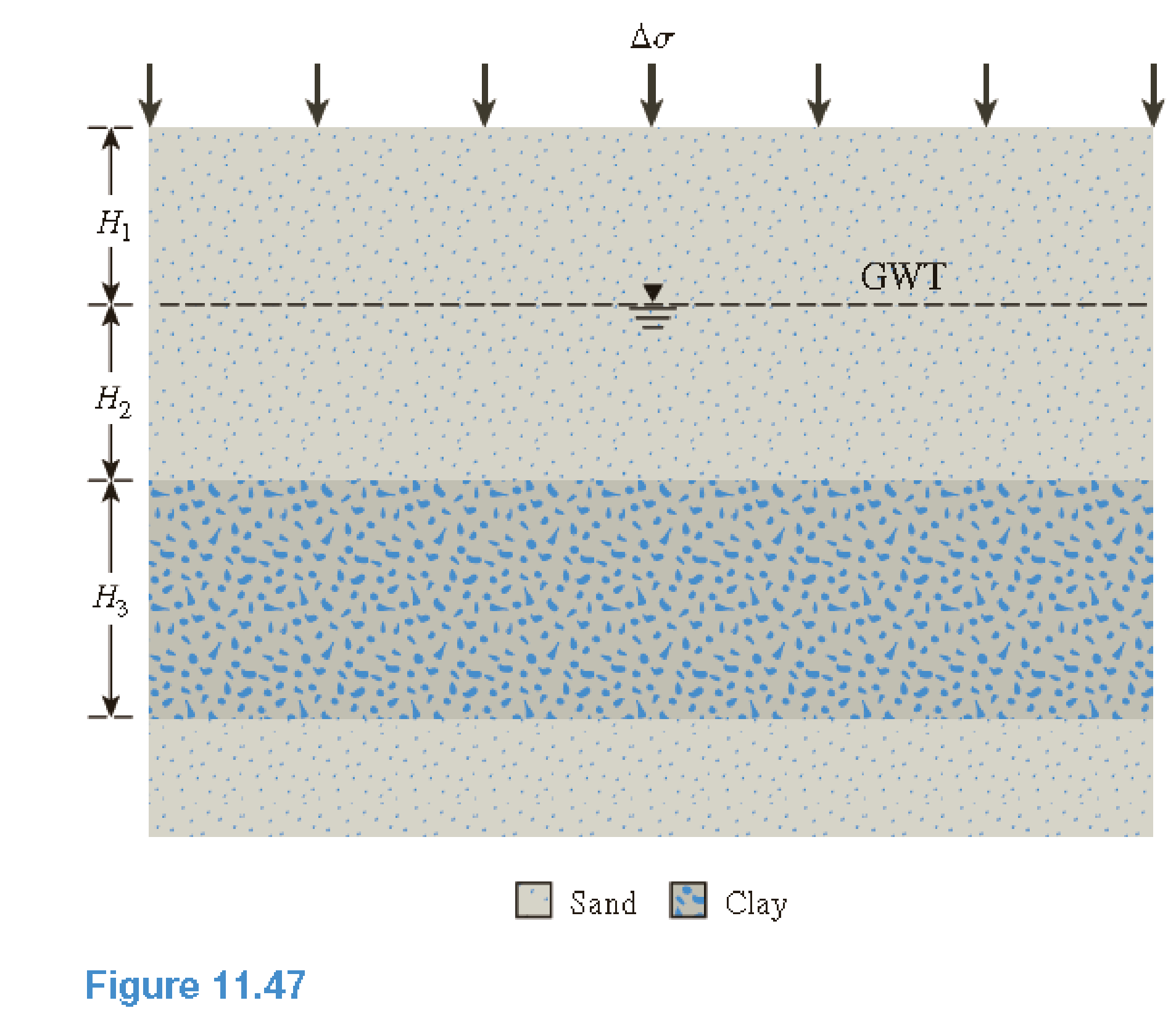Chapter 11, Problem 11.11PPrinciples of Geotechnical Enginee...

9th Edition
Braja M. Das + 1 other
ISBN: 9781305970939

Solutions

Chapter
SectionPrinciples of Geotechnical Enginee...

9th Edition
Braja M. Das + 1 other
ISBN: 9781305970939
Textbook Problem

Refer to Figure 11.47. Estimate the primary consolidation settlement in the clay layer. Given: Δ σ = 105   kN / m 2 , H 1 = 2.2   m , H 2 = 4.4   m , and H 3 = 7.5   m . Soil characteristics areSand: e = 0.58; Gs = 2.67Clay: LL = 49; e = 1.08; Gs = 2.71; σ ′ c = 210   kN / m 2 ; C s = 1 6 C cTo determine

Calculate the primary consolidation settlement of the clay layer.

Explanation

Given information:

The net applied pressure (Δσ) is 105kN/m2.

The height of the sand layer 1 (H1) is 2.2m.

The height of the sand layer 2 (H2) is 4.4m.

The height of the clay layer 3 (H3) is 7.5m.

The void ratio of sand (e) is 0.58.

The specific gravity of soil solids for sand (Gs) is 2.67.

The void ratio of clay (e) is 1.08.

The specific gravity of soil solids for clay (Gs) is 2.71.

The liquid limit (LL) is 49.

The preconsolidation pressure (σc) is 210kN/m2.

The swell index (CS) is 16CC.

Calculation:

Consider the unit weight of water (γw) is 9.81kN/m3.

Calculate the dry unit weight of sand (γd(sand)) using the relation.

γd(sand)=Gsγw1+e

Substitute 2.67 for Gs, 9.81kN/m3 for γw, and 0.58 for e.

γd(sand)=2.67×9.811+0.58=26.191.58=16.57kN/m3

Calculate the saturated unit weight of sand (γ(sand)) using the relation.

γ(sand)=(Gs1)γw1+e

Substitute 2.67 for Gs, 9.81kN/m3 for γw, and 0.58 for e.

γ(sand)=(2.671)×9.811+0.58=16.381.58=10.36kN/m3

Calculate the saturated unit weight of clay (γ(sand)) using the relation.

γ(clay)=(Gs1)γw1+e

Substitute 2.71 for Gs, 9.81kN/m3 for γw, and 1.08 for e.

γ(clay)=(2.711)×9.811+1.08=16.7752.08=8.06kN/m3

Calculate the compression index (CC) using the relation.

CC=0.009(LL10)

Substitute 49 for LL.

CC=0.009(4910)=0.351

Calculate the swell index (CS) using the relation as shown below.

CS=16CC

Substitute 0

Still sussing out bartleby?

Check out a sample textbook solution.

See a sample solution

The Solution to Your Study Problems

Bartleby provides explanations to thousands of textbook problems written by our experts, many with advanced degrees!

Get Started

Describe how a sub-spindle can be used to increase productivity.

Precision Machining Technology (MindTap Course List)

What is a DBMS, and what are its functions?

Database Systems: Design, Implementation, & Management

What is COSO, and why is it important?

Management Of Information Security

Define a use case and a use case diagram, and prepare a sample of each.

Systems Analysis and Design (Shelly Cashman Series) (MindTap Course List)

Explain optoisolation.

Electric Motor Control

Why is it important to seek medical treatment for eye burns?

Welding: Principles and Applications (MindTap Course List)

What is a service pack?

Enhanced Discovering Computers 2017 (Shelly Cashman Series) (MindTap Course List)